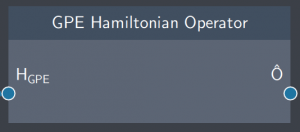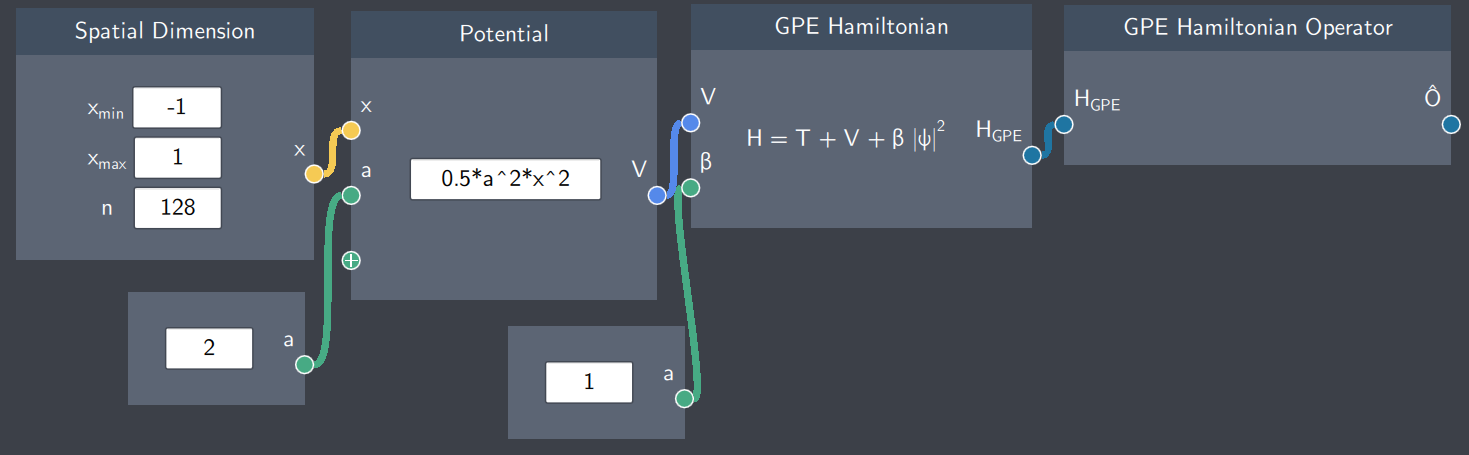# GPE Hamiltonian Operator

## Description

The GPE Hamiltonian Operator node changes the GPE Hamiltonian to the corresponding quantum mechanical operator.## Input

The node has the following input:

• GPE Hamiltionian ($H_{GPE}$): This input comes from the GPE Hamiltonian node

## Content

The node converts the GPE Hamiltonian to a operator that can be applied to a state.

## Output

The node outputs the GPE Hamiltonian as an operator which can be applied to a state.

## Example

In the example below, the GPE Hamiltonian operator takes the GPE Hamiltonian as the input. The complete connections between the nodes starting from the spatial dimensions up to the operator are shown below.